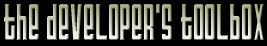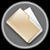This section of the archives stores flipcode's complete Developer Toolbox collection, featuring a variety of mini-articles and source code contributions from our readers.Object To Screen Space   Submitted byThe OpenGL pipeline automatically transforms object space vertices to homogeneous clip space and then to screen space-- pixel coordinates and a z-buffer value betwen 0 and 1. When combining 2D and 3D rendering, you need to perform that transformation manually. Two example applications are finding the 3D ray corresponding to a 2D mouse position and the location at which to render 2D text over 3D vertices. The glToScreen function listed below transform an object space point to screen space using the current GL_MODELVIEW and GL_PROJECTION matrices. The resulting xy values are in pixels, the z value is on the glDepthRange scale, which defaults to [0, 1]), and the w value contains rhw. The rhw value is -1/z for camera space z (note that a true mathematical projection gives w = 1 instead.) The rhw value is useful for scaling line and point size with perspective, which OpenGL does not do by default. Morgan McGuire

 ```Vector4 glToScreen(const Vector4& v) { // Get the matrices and viewport double modelView; double projection; double viewport; double depthRange; glGetDoublev(GL_MODELVIEW_MATRIX, modelView); glGetDoublev(GL_PROJECTION_MATRIX, projection); glGetDoublev(GL_VIEWPORT, viewport); glGetDoublev(GL_DEPTH_RANGE, depthRange); // Compose the matrices into a single row-major transformation Vector4 T; int r, c, i; for (r = 0; r < 4; ++r) { for (c = 0; c < 4; ++c) { T[r][c] = 0; for (i = 0; i < 4; ++i) { // OpenGL matrices are column major T[r][c] += projection[r + i * 4] * modelView[i + c * 4]; } } } // Transform the vertex Vector4 result; for (r = 0; r < 4; ++r) { result[r] = T[r].dot(v); } // Homogeneous divide const double rhw = 1 / result.w; return Vector4( (1 + result.x * rhw) * viewport / 2 + viewport, (1 - result.y * rhw) * viewport / 2 + viewport, (result.z * rhw) * (depthRange - depthRange) + depthRange, rhw); } ```